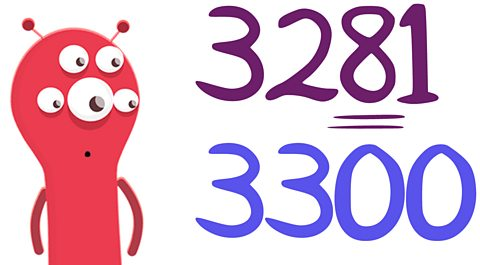# Monthly Archives: May 2018

This week we have been learning about squared and cubed numbers in Maths, which was fantastic! We also learnt about time zones and why we have them. Today we created an information text about everything we have learnt so far on space, including how the planets orbit the sun.

This week’s attendance is 98.7%.

# Maths Support Week 1 Summer 2

The first week back after half term, the children will be focussing on place value. The children will round any number up to 1,000,000 to the nearest 10, 100, 1000, 10000 or 100000.

### Rounding to the nearest 100

To round a number to the nearest 100, look at the tens digit. If the tens digit is 5 or more, round up. If the tens digit is 4 or less, round down.

• The tens digit in 3281 is 8. So we round it up to 3300.
• The tens digit of 3216 is 1. So we round it down to 3200When rounding to the nearest 100, 3281 becomes 3300.

### Rounding to the nearest 1000

To round a number to the nearest 1000, look at the hundreds digit. If the hundreds digit is 5 or more, round up. If the hundreds digit is 4 or less, round down.

• The hundreds digit in 4559 is 5. So we round it up to 5000.
• The hundreds digit of 4295 is 2. So we round it down to 4000.

WHAT WE’VE LEARNT THIS WEEK !

This week in English we have been planning a story narrative based on the book of cosmic ( when Liam and the crew were trapped in the deep,dark shadows of  space ,their only mission was to get back home to earth) .

In math this week , we have been doing multi-step problems involving addition, subtraction, fluency and reasoning questions, which i have found challenging but fun .

This week we have been taking lessons in the year 6 classroom as they have been doing their SATS .

In PE this week we have been doing the triple  jump ( with Mr Willock ) and with Mr Carr we have been doing rounders which i found exciting and fun .

In science this week we have been  learning about space and how the planets rotate around the sun . (orbit)

Attendance this week is 97.7%

thanks Holly

# Maths Support Week 6

In the final week of this half term, the children will be recognising and using square numbers and cube numbers, and the notation for square2 and cubed3.

Square numbers

A square number is a number multiplied by itself. This can also be called ‘a number squared’. The symbol for squared is ².

2² = 2 x 2 = 4

3² = 3 x 3 = 9

4² = 4 x 4 = 16

5² = 5 x 5 = 25

The square numbers up to 100 are: 1, 4, 9, 16, 25, 36, 49, 64, 81, 100.

Cube numbers

A cube number is a number multiplied by itself 3 times. This can also be called ‘a number cubed’.The symbol for cubed is ³.

2³ = 2 × 2 × 2 = 8

3³ = 3 × 3 × 3 = 27

4³ = 4 × 4 × 4 = 64

5³ = 5 × 5 × 5 =125

The cube numbers up to 100 are: 1, 8, 27, 64

# What We Have Been Learning About!

This week we have been learning about rounding. We have rounded decimal numbers up to 2 decimal places, as well as rounding amounts of money. In science, we have been looking at whether the Earth is flat or spherical. After gathering lots of different theories and evidence together, it’s apparent that the Earth is in fact a sphere.

Class attendance-99.8%

Ethan.

# Maths Support Week 5

Next week the children will be tackling multistep problems using addition and subtraction. The children will mainly be looking at money problems where they have to show their workings out using the correct method.

Useful tips for solving money problems:

• Read the words of the problem carefully to decide whether to use adding, subtracting, multiplying or dividing.
• If some of the prices in the problem are in pence and some are in pounds, change some of them so they are either ALL in pounds or ALL in pence.
• Treat money problems just like normal number calculations, but remember to put the decimal point and pound symbol in the right place.

## Example 1

You buy a talking robot for £9.87 and a magazine for 73p. How much will you spend altogether?

1. First make sure both amounts are in the same units. 73p = £0.73.
2. Then add the two amounts by lining up the decimal points.3. So the total you will spend is £10.60. (If you had worked this out on a calculator, you would have got 10.6. Remember to write this as £10.60.)

## Example 2

You can buy a 4-can pack of lemonade for £1.00 or individual cans for 28p. Which is better value for money?

1. Work out how much one can in the 4-can pack costs by dividing the price by the number of cans. £1.00 ÷ 4 = £0.25 or 25p.
2. So the 4-can pack is better value because each can costs 25p, that is 3p cheaper than individual cans.